bpf
Compute and display the band-pass filter of a series.
Computes, and displays a graphical view of the Baxter-King fixed length symmetric, Christiano-Fitzgerald fixed length symmetric, or the Christiano-Fitzgerald full sample asymmetric band-pass filter of the series.
The view will show the original series, the cyclical component, and non-cyclical component in a single graph. For non time-varying filters, a second graph will show the frequency responses.
Syntax
series_name.bpf(options) [cyc_name]
Follow the bpf keyword with any desired options, and the optional name to be given to the cyclical component. If you do not provide cyc_name, the filtered series will be named BPFILTER## where ## is a number chosen to ensure that the name is unique.
To display the graph, you may need to precede the object command with the “show” keyword.
Options

 type=arg (default=“bk”) Specify the type of band-pass filter: “bk” is the Baxter-King fixed length symmetric filter, “cffix” is the Christiano-Fitzgerald fixed length symmetric filter, “cfasym” is the Christiano-Fitzgerald full sample asymmetric filter. low=number, high=number Low (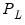) and high (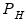) values for the cycle range to be passed through (specified in periods of the workfile frequency). Defaults to the workfile equivalent corresponding to a range of 1.5–8 years for semi-annual to daily workfiles; otherwise sets “low=2”, “high=8”.The arguments must satisfy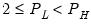. The corresponding frequency range to be passed through will be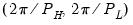. lag=integer Fixed lag length (positive integer). Sets the fixed lead/lag length for fixed length filters (“type=bk” or “type=cffix”). Must be less than half the sample size. Defaults to the workfile equivalent of 3 years for semi-annual to daily workfiles; otherwise sets “lag=3”. iorder=[0,1] (default=0) Specifies the integration order of the series. The default value, “0” implies that the series is assumed to be (covariance) stationary; “1” implies that the series contains a unit root. The integration order is only used in the computation of Christiano-Fitzgerald filter weights (“type=cffix” or “type=cfasym”). When “iorder=1”, the filter weights are constrained to sum to zero. detrend=arg (default=“n”) Detrending method for Christiano-Fitzgerald filters (“type=cffix” or “type=cfasym”). You may select the default argument “n” for no detrending, “c” to demean, or “t” to remove a constant and linear trend.You may use the argument “d” to remove drift, if the option “iorder=1” is also specified. nogain Suppresses plotting of the frequency response (gain) function for fixed length symmetric filters (“type=bk” or “type=cffix”). By default, EViews will plot the gain function. noncyc=arg Specifies a name for a series to contain the non-cyclical series (difference between the actual and the filtered series). If no name is provided, the non-cyclical series will not be saved in the workfile. w=arg Store the filter weights as an object with the specified name. For fixed length symmetric filters (“type=bk” or “type=cffix”), the saved object will be a matrix of dimension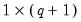where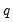is the user-specified lag length order. For these filters, the weights on the leads and the lags are the same, so the returned matrix contains only the one-sided weights. The filtered series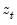may be computed as: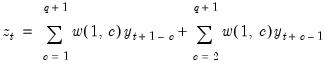for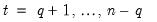.For time-varying filters, the weight matrix is of dimension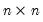where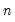is the number of non-missing observations in the current sample. Row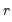of the matrix contains the weighting vector used to generate the-th observation of the filtered series, where column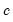contains the weight on the-th observation of the original series. The filtered series may be computed as: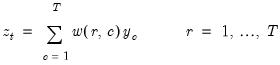where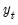is the original series and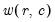is the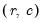element of the weighting matrix. By construction, the first and last rows of the weight matrix will be filled with missing values for the symmetric filter. prompt Force the dialog to appear from within a program. p Print the graph.
Examples
Suppose we are working in a quarterly workfile and we issue the following command:
lgdp.bpf(type=bk,low=6,high=32) cyc0
EViews will compute the Baxter-King band-pass filter of the series LGDP. The periodicity of cycles extracted ranges from 6 to 32 quarters, and the filtered series will be saved in the workfile in CYC0. The BK filter uses the default lag of 12 (3 years of quarterly data).
Since this is a fixed length filter, EViews will display both a graph of the cyclical/original/non-cyclical series, as well as the frequency response (gain) graph. To suppress the latter graph, we could enter a command containing the “nogain” option:
lgdp.bpf(type=bk,low=6,high=32,lag=12,nogain)
In this example, we have also overridden the default by specifying a fixed lag of 12 (quarters). Since we have omitted the name for the cyclical series, EViews will create a series with a name like BPFILTER01 to hold the results.
To compute the asymmetric Christiano-Fitzgerald filter, we might enter a command of the form:
lgdp.bpf(type=cfasym,low=6,high=32,noncyc=non1,weight=wm) cyc0
The cyclical components are saved in CYC0, the non-cyclical in NON1, and the weighting matrix in WM.
Cross-references